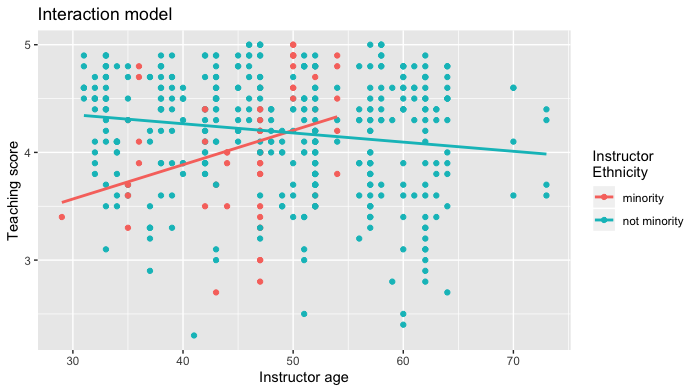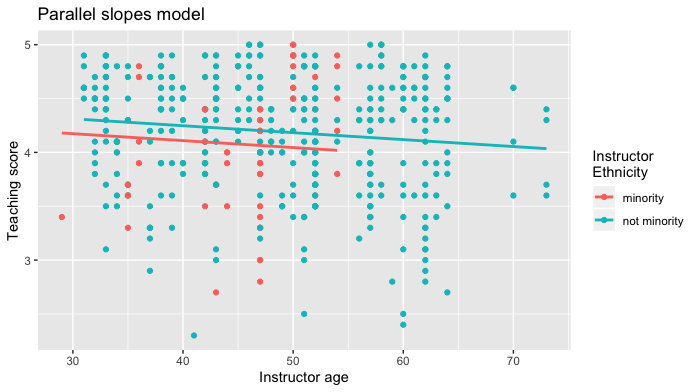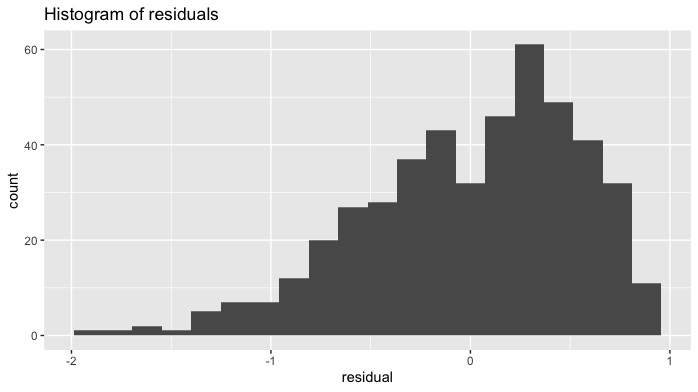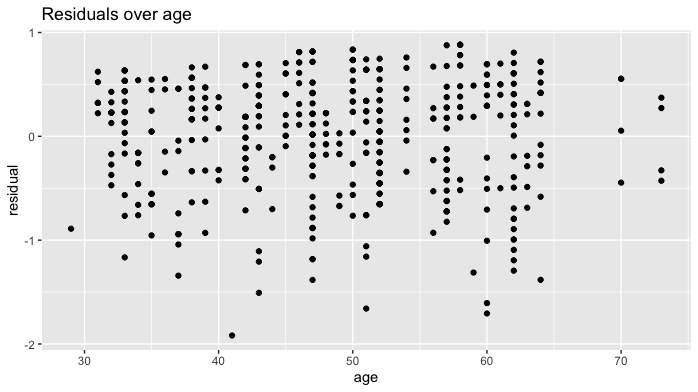# Why should you use the moderndive package for intro linear regression?

## Intro

Linear regression has long been a staple of introductory statistics courses. While the timing of when to introduce it may have changed (many argue that descriptive regression should be done ASAP and then revisited later after statistical inference has been covered), it’s overall importance in the intro stats curriculum remains the same.

Let’s consider data gathered from end of semester student evaluations for 463 professors from the University of Texas at Austin (see openintro.org for more details). Here is a random sample of 5 instructors and a subset of 8 of the 13 variables included, where the outcome variable of interest is the teaching evaluation `score` out of 5 as given by students:

ID score age bty_avg gender ethnicity language rank
129 3.7 62 3.000 male not minority english tenured
109 4.7 46 4.333 female not minority english tenured
28 4.8 62 5.500 male not minority english tenured
434 2.8 62 2.000 male not minority english tenured
330 4.0 64 2.333 male not minority english tenured

The data is included in `evals` dataset in the `moderndive` R package for tidyverse-friendly introductory linear regression. Let’s fit a regression model of teaching `score` as a function of instructor `age`:

``````library(moderndive)
score_model <- lm(score ~ age, data = evals)``````

### Regression analysis the “good old-fashioned” way

Let’s analyze the output of the fitted model `score_model` “the good old fashioned way”: using `summary.lm()`.

``````summary(score_model)
#>
#> Call:
#> lm(formula = score ~ age, data = evals)
#>
#> Residuals:
#>     Min      1Q  Median      3Q     Max
#> -1.9185 -0.3531  0.1172  0.4172  0.8825
#>
#> Coefficients:
#>              Estimate Std. Error t value Pr(>|t|)
#> (Intercept)  4.461932   0.126778  35.195   <2e-16 ***
#> age         -0.005938   0.002569  -2.311   0.0213 *
#> ---
#> Signif. codes:  0 '***' 0.001 '**' 0.01 '*' 0.05 '.' 0.1 ' ' 1
#>
#> Residual standard error: 0.5413 on 461 degrees of freedom
#> Multiple R-squared:  0.01146,    Adjusted R-squared:  0.009311
#> F-statistic: 5.342 on 1 and 461 DF,  p-value: 0.02125``````

Here are five common student comments/questions we’ve heard over the years in our intro stats courses based on this output:

1. “Wow! Look at those p-value stars! Stars are good, so I must get more stars somehow.”
2. “How do extract the values in the regression table?”
3. “Where are the fitted/predicted values and residuals?”
4. “How do I apply this model to a new set of data to make predictions?”
5. “What is all this other stuff at the bottom?”

### Regression analysis the tidyverse-friendly way

To address these comments/questions, we’ve included three functions in the `moderndive` package that takes as a fitted model as input (in this case `score_model`) and returns the same information as `summary(score_model)` but in a tidyverse-friendly way:

1. Get a tidy regression table with confidence intervals:

``````get_regression_table(score_model)
#> # A tibble: 2 x 7
#>   term      estimate std_error statistic p_value lower_ci upper_ci
#>   <chr>        <dbl>     <dbl>     <dbl>   <dbl>    <dbl>    <dbl>
#> 1 intercept    4.46      0.127     35.2    0        4.21     4.71
#> 2 age         -0.006     0.003     -2.31   0.021   -0.011   -0.001``````
2. Get information on each point/observation in your regression, including fitted/predicted values & residuals, in a single data frame:

``````get_regression_points(score_model)
#> # A tibble: 463 x 5
#>       ID score   age score_hat residual
#>    <int> <dbl> <int>     <dbl>    <dbl>
#>  1     1   4.7    36      4.25    0.452
#>  2     2   4.1    36      4.25   -0.148
#>  3     3   3.9    36      4.25   -0.348
#>  4     4   4.8    36      4.25    0.552
#>  5     5   4.6    59      4.11    0.488
#>  6     6   4.3    59      4.11    0.188
#>  7     7   2.8    59      4.11   -1.31
#>  8     8   4.1    51      4.16   -0.059
#>  9     9   3.4    51      4.16   -0.759
#> 10    10   4.5    40      4.22    0.276
#> # … with 453 more rows``````
3. Get scalar summaries of a regression fit including \(R^2\) and \(R^2_{adj}\) but also the (root) mean-squared error:

``````get_regression_summaries(score_model)
#> # A tibble: 1 x 8
#>   r_squared adj_r_squared   mse  rmse sigma statistic p_value    df
#>       <dbl>         <dbl> <dbl> <dbl> <dbl>     <dbl>   <dbl> <dbl>
#> 1     0.011         0.009 0.292 0.540 0.541      5.34   0.021     2``````

### Visualizing parallel slopes models

Say you are plotting a scatterplot with a categorical variable mapped to the `color` aesthetic. Using `geom_smooth(method = "lm", se = FALSE)` from the `ggplot2` package yields a visualization of an interaction model (different intercepts and different slopes):

``````library(ggplot2)
ggplot(evals, aes(x = age, y = score, color = ethnicity)) +
geom_point() +
labs(x = "Instructor age", y = "Teaching score", color = "Instructor\nEthnicity",
title = "Interaction model") +
geom_smooth(method = "lm", se = FALSE)``````However, say you want to plot a parallel slopes model. For example, many introductory statistics textbooks start with the simpler-to-teach “common slope, different intercepts” regression model. Alas however, you can’t visualize such models using `geom_smooth()`.

Evgeni Chasnovski thus wrote a custom `geom_` extension to `ggplot2` called `geom_parallel_slopes()`. You use it just as you would any `geom_`etric object in `ggplot2`, but you need to have the `moderndive` package loaded as well:

``````library(ggplot2)
library(moderndive)
ggplot(evals, aes(x = age, y = score, color = ethnicity)) +
geom_point() +
labs(x = "Instructor age", y = "Teaching score", color = "Instructor\nEthnicity",
title = "Parallel slopes model") +
geom_parallel_slopes(se = FALSE)``````At this point however, students will inevitably ask a sixth question: “When would you ever use a parallel slopes model?”

### Why should you use the `moderndive` package?

We think that these functions included in the `moderndive` package are effective pedagogical tools that can help address the above six common student comments/questions. We now argue why.

## 1. Less p-value stars, more confidence intervals

The first common student comment/question:

“Wow! Look at those p-value stars! Stars are good, so I must get more stars somehow.”

We argue that the `summary()` output is deficient in an intro stats setting because:

• The `Signif. codes: 0 '***' 0.001 '**' 0.01 '*' 0.05 '.' 0.1 ' ' 1` only encourage p-hacking. In case you have not yet been convinced of the perniciousness of p-hacking, perhaps comedian John Oliver can convince you.
• While not a silver bullet for eliminating misinterpretations of statistical inference results, confidence intervals at least give students a sense of the practical significance and not just the statistical significance of the results. These should be included in the regression table output.

Instead of `summary()`, let’s use the `get_regression_table()` function in the `moderndive` package:

``````get_regression_table(score_model)
#> # A tibble: 2 x 7
#>   term      estimate std_error statistic p_value lower_ci upper_ci
#>   <chr>        <dbl>     <dbl>     <dbl>   <dbl>    <dbl>    <dbl>
#> 1 intercept    4.46      0.127     35.2    0        4.21     4.71
#> 2 age         -0.006     0.003     -2.31   0.021   -0.011   -0.001``````

Confidence intervals! By including them in the output, we can easily emphasize to students that they “surround” the point estimates in the `estimate` column. Note the confidence level is defaulted to 95%.

## 2. Outputs as tidy tibbles!

All the functions in the `moderndive` package return tidy tibbles! So for example, by piping the above `get_regression_table(score_model)` output into the `kable()` function from the `knitr` package, you can have aesthetically pleasing regression tables in R Markdown documents, instead of jarring computer output font:

``````get_regression_table(score_model) %>%
knitr::kable()``````
term estimate std_error statistic p_value lower_ci upper_ci
intercept 4.462 0.127 35.195 0.000 4.213 4.711
age -0.006 0.003 -2.311 0.021 -0.011 -0.001

Now let’s address the second common student comment/question:

“How do extract the values in the regression table?”

While one might argue that extracting the intercept and slope coefficients can be simply done using `coefficients(score_model)`, what about the standard errors? A Google query of “how do I extract standard errors from lm in r” yields results from the R mailing list and from crossvalidated suggesting we run:

``````sqrt(diag(vcov(score_model)))
#> (Intercept)         age
#> 0.126778499 0.002569157``````

Say what?!? It shouldn’t be this hard! However since `get_regression_table()` returns a data frame/tidy tibble, you can easily extract columns using `dplyr::pull()`:

``````get_regression_table(score_model) %>%
pull(std_error)
#>  0.127 0.003``````

or equivalently you can use the `\$` sign operator from base R:

``````get_regression_table(score_model)\$std_error
#>  0.127 0.003``````

## 3. Birds of a feather should flock together: Fitted values & residuals

The third common student comment/question:

“Where are the fitted/predicted values and residuals?”

How can we extract point-by-point information from a regression model, such as the fitted/predicted values and the residuals? (Note we’ll only display the first 10 of such values, and not all n = 463, for brevity’s sake)

``fitted(score_model)``
``````#>        1        2        3        4        5        6        7        8
#> 4.248156 4.248156 4.248156 4.248156 4.111577 4.111577 4.111577 4.159083
#>        9       10
#> 4.159083 4.224403``````
``residuals(score_model)``
``````#>           1           2           3           4           5           6
#>  0.45184376 -0.14815624 -0.34815624  0.55184376  0.48842294  0.18842294
#>           7           8           9          10
#> -1.31157706 -0.05908286 -0.75908286  0.27559666``````

But why have the original explanatory/predictor `age` and outcome variable `score` in `evals`, the fitted/predicted values `score_hat`, and `residual` floating around in separate pieces? Since each observation relates to the same instructor, wouldn’t it make sense to organize them together? This is where `get_regression_points()` shines!

``get_regression_points(score_model)``
``````#> # A tibble: 10 x 5
#>       ID score   age score_hat residual
#>    <int> <dbl> <int>     <dbl>    <dbl>
#>  1     1   4.7    36      4.25    0.452
#>  2     2   4.1    36      4.25   -0.148
#>  3     3   3.9    36      4.25   -0.348
#>  4     4   4.8    36      4.25    0.552
#>  5     5   4.6    59      4.11    0.488
#>  6     6   4.3    59      4.11    0.188
#>  7     7   2.8    59      4.11   -1.31
#>  8     8   4.1    51      4.16   -0.059
#>  9     9   3.4    51      4.16   -0.759
#> 10    10   4.5    40      4.22    0.276``````

Observe that the original outcome variable `score` and explanatory/predictor variable `age` are now supplemented with the fitted/predicted value `score_hat` and `residual` columns. By putting the fitted/predicted values and the residuals next to the original data, we argue that the computation of these values is less opaque. For example in class, instructors can write out by hand how all the values in the first row, corresponding to the first instructor, are computed.

Furthermore, recall that all outputs in the `moderndive` package are data frames/tidy tibbles, thus you can easily create custom residual analysis plots, instead of the default ones yielded by `plot(score_model)`. For example, we create:

• A histogram of all residuals to investigate normality.
• A partial residual plot of the relationship of the residuals vs all explanatory/predictor variables to investigate the presence of heteroskedasticity; in this case a scatterplot of the residuals over `age`.
``````score_model_points <- get_regression_points(score_model)

# Histogram of residuals:
ggplot(score_model_points, aes(x = residual)) +
geom_histogram(bins = 20) +
labs(title = "Histogram of residuals")````````````
# Investigating patterns:
ggplot(score_model_points, aes(x = age, y = residual)) +
geom_point() +
labs(title = "Residuals over age")``````## 4. Baby’s first Kaggle predictive modeling competition submission!

The fourth common student comment/question:

“How do I apply this model to a new set of data to make predictions?”

With the fields of machine learning and artificial intelligence gaining prominence, the importance of predictive modeling cannot be understated. Therefore, we’ve designed the `get_regression_points()` function to allow for a `newdata` argument to quickly apply a previously fitted model to “new” observations.

Let’s create an artificial “new” dataset which is a subset of the original `evals` data with the outcome variable `score` removed and use it as the `newdata` argument:

``````new_evals <- evals %>%
sample_n(4) %>%
select(-score)
new_evals
#> # A tibble: 4 x 13
#>      ID prof_ID   age bty_avg gender ethnicity language rank  pic_outfit
#>   <int>   <int> <int>   <dbl> <fct>  <fct>     <fct>    <fct> <fct>
#> 1   272      51    57    5.67 male   not mino… english  tenu… not formal
#> 2   239      45    33    7    male   not mino… english  tenu… formal
#> 3    87      16    45    4.17 male   not mino… english  tenu… not formal
#> 4   108      19    46    4.33 female not mino… english  tenu… not formal
#> # … with 4 more variables: pic_color <fct>, cls_did_eval <int>,
#> #   cls_students <int>, cls_level <fct>

get_regression_points(score_model, newdata = new_evals)
#> # A tibble: 4 x 3
#>      ID   age score_hat
#>   <int> <int>     <dbl>
#> 1     1    57      4.12
#> 2     2    33      4.27
#> 3     3    45      4.20
#> 4     4    46      4.19``````

`score_hat` are the predicted values! Let’s do another example, this time using the Kaggle House Prices: Advanced Regression Techniques practice competition.